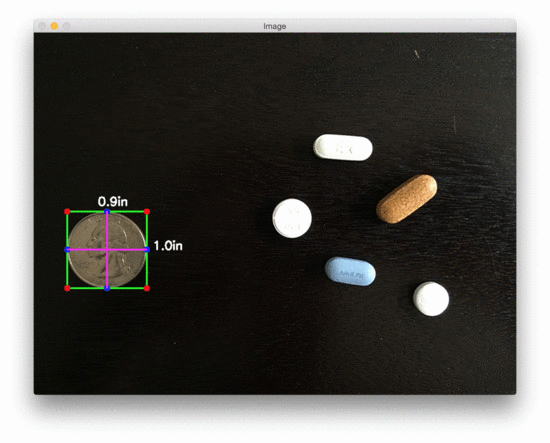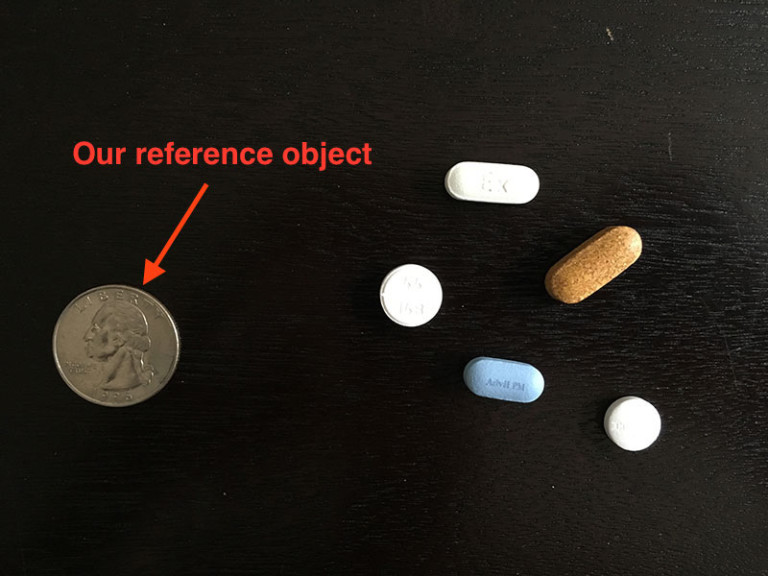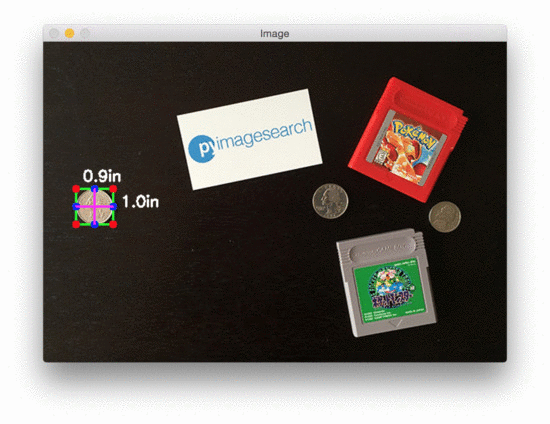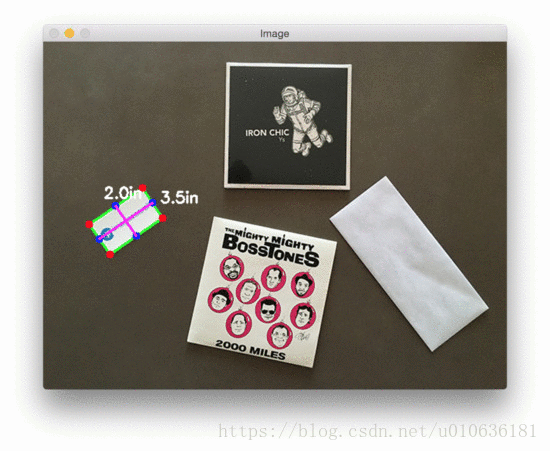# 使用OpenCV测量图像中物体的大小## pixels_per_metric

1. 我们应该知道这个物体的真实尺寸（在宽度或高度上的毫米或英寸等值的大小）。
2. 我们应该能够轻松地在图片中找到这个参照物，要么基于参照物的位置(如，参照物可以是一副图像中左上角的物体)或基于参照物的外表(例如参照物可以是图片中具有最独特的颜色或独一无二的形状，不同于所有其他的物体)。
在任何一种情况下，我们的参考应该以某种方式唯一可识别。pixels_per_metric =物体像素宽 / 物体真实宽

pixels_per_metric = 150px / 0.955in = 157px

## 利用计算机视觉测量物体大小

# import the necessary packages
from scipy.spatial import distance as dist
from imutils import perspective
from imutils import contours
import numpy as np
import argparse
import imutils
import cv2

def midpoint(ptA, ptB):
return ((ptA + ptB) * 0.5, (ptA + ptB) * 0.5)

# construct the argument parse and parse the arguments
ap = argparse.ArgumentParser()
ap.add_argument("-i", "--image", required=True,
help="path to the input image")
ap.add_argument("-w", "--width", type=float, required=True,
help="width of the left-most object in the image (in inches)")
args = vars(ap.parse_args())

$pip install imutils 另外如果你已经安装过这个imutils包，也请确保它为最新版本，在我这里所使用最新的版本是0.3.6。 $ pip install --upgrade imutils

# load the image, convert it to grayscale, and blur it slightly
image = cv2.imread("F:\py\example_03.jpg")
gray = cv2.cvtColor(image, cv2.COLOR_BGR2GRAY)
gray = cv2.GaussianBlur(gray, (7, 7), 0)

# perform edge detection, then perform a dilation + erosion to
# close gaps in between object edges
edged = cv2.Canny(gray, 50, 100)
edged = cv2.dilate(edged, None, iterations=1)
edged = cv2.erode(edged, None, iterations=1)

# find contours in the edge map
cnts = cv2.findContours(edged.copy(), cv2.RETR_EXTERNAL,
cv2.CHAIN_APPROX_SIMPLE)
cnts = cnts if imutils.is_cv2() else cnts

# sort the contours from left-to-right and initialize the
# 'pixels per metric' calibration variable
(cnts, _) = contours.sort_contours(cnts)
pixelsPerMetric = None

# loop over the contours individually
for c in cnts:
# if the contour is not sufficiently large, ignore it
if cv2.contourArea(c) < 100:
continue

# compute the rotated bounding box of the contour
orig = image.copy()
box = cv2.minAreaRect(c)
box = cv2.cv.BoxPoints(box) if imutils.is_cv2() else cv2.boxPoints(box)
box = np.array(box, dtype="int")

# order the points in the contour such that they appear
# in top-left, top-right, bottom-right, and bottom-left
# order, then draw the outline of the rotated bounding
# box
box = perspective.order_points(box)
cv2.drawContours(orig, [box.astype("int")], -1, (0, 255, 0), 2)

# loop over the original points and draw them
for (x, y) in box:
cv2.circle(orig, (int(x), int(y)), 5, (0, 0, 255), -1)

    # unpack the ordered bounding box, then compute the midpoint
# between the top-left and top-right coordinates, followed by
# the midpoint between bottom-left and bottom-right coordinates
(tl, tr, br, bl) = box
(tltrX, tltrY) = midpoint(tl, tr)
(blbrX, blbrY) = midpoint(bl, br)

# compute the midpoint between the top-left and top-right points,
# followed by the midpoint between the top-righ and bottom-right
(tlblX, tlblY) = midpoint(tl, bl)
(trbrX, trbrY) = midpoint(tr, br)

# draw the midpoints on the image
cv2.circle(orig, (int(tltrX), int(tltrY)), 5, (255, 0, 0), -1)
cv2.circle(orig, (int(blbrX), int(blbrY)), 5, (255, 0, 0), -1)
cv2.circle(orig, (int(tlblX), int(tlblY)), 5, (255, 0, 0), -1)
cv2.circle(orig, (int(trbrX), int(trbrY)), 5, (255, 0, 0), -1)

# draw lines between the midpoints
cv2.line(orig, (int(tltrX), int(tltrY)), (int(blbrX), int(blbrY)),
(255, 0, 255), 2)
cv2.line(orig, (int(tlblX), int(tlblY)), (int(trbrX), int(trbrY)),
(255, 0, 255), 2)

此外，我们还分别计算左上角与左下角，右上角和右下角的中点（第10和11行）。

第14-17行在我们的图像上画出蓝色的中点，然后将各中间点用紫色线连接起来。

接下来，我们需要通过查看我们的参照物来初始化pixelsPerMetric变量：

    # compute the Euclidean distance between the midpoints
dA = dist.euclidean((tltrX, tltrY), (blbrX, blbrY))
dB = dist.euclidean((tlblX, tlblY), (trbrX, trbrY))

# if the pixels per metric has not been initialized, then
# compute it as the ratio of pixels to supplied metric
# (in this case, inches)
if pixelsPerMetric is None:
pixelsPerMetric = dB / args["width"]

dA变量将包含高度距离（以像素为单位），而dB将保持我们的宽度距离。

    # compute the size of the object
dimA = dA / pixelsPerMetric
dimB = dB / pixelsPerMetric

# draw the object sizes on the image
cv2.putText(orig, "{:.1f}in".format(dimA),
(int(tltrX - 15), int(tltrY - 10)), cv2.FONT_HERSHEY_SIMPLEX,
0.65, (255, 255, 255), 2)
cv2.putText(orig, "{:.1f}in".format(dimB),
(int(trbrX + 10), int(trbrY)), cv2.FONT_HERSHEY_SIMPLEX,
0.65, (255, 255, 255), 2)

# show the output image
cv2.imshow("Image", orig)
cv2.waitKey(0)

## 物体大小测试结果

 python size.py --image images/example_01.jpg --width 0.955首先，我匆忙用我的iPhone拍了这张照片。这个角度当然不是一个完美的90度角“向下看”（就像鸟的眼睛一样）在物体上。如果没有一个完美的90度视图（或者尽可能接近它），物体的尺寸就会被扭曲。

其次，我没有使用相机的内在和外在参数来校准我的iPhone。如果不确定这些参数，照片就会倾向于径向和切向镜头失真。执行一个额外的校准步骤来发现这些参数可以“不扭曲”我们的图像，并导致更好的对象大小近似（但是我将把关于失真校正的讨论作为未来博客文章的主题）。

换句话说，在拍摄对象的照片时，尽量接近90度的视角——这将有助于提高对物体大小估计的准确性。

让我们看第二个测量物体大小的例子，这次测量的是药片的尺寸：

python size.py --image images/example_02.jpg --width 0.955https://blog.csdn.net/u010636181/article/details/80659700

03-0653806-124万+
12-022万+
08-01924
06-108851
10-151356
01-233793
04-039404
05-292万+
08-172万+
01-12305
05-011万+
12-22433
©️2020 CSDN 皮肤主题: 大白 设计师:CSDN官方博客